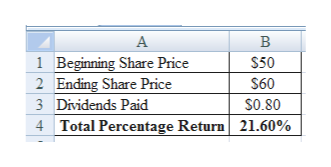# Suppose a stock had an initial price of \$50 per share, paid a dividend of \$.80 per share during the year, and had an ending share price of \$60. Compute the total percentage return. (Do not round intermediate calculations. Enter your answer as a percent rounded to 2 decimal places, e.g., 32.16.) Total return             %

Question
2 views

Suppose a stock had an initial price of \$50 per share, paid a dividend of \$.80 per share during the year, and had an ending share price of \$60.

Compute the total percentage return. (Do not round intermediate calculations. Enter your answer as a percent rounded to 2 decimal places, e.g., 32.16.)

Total return             %

check_circle

Step 1

Calculation of Total Percentage Return:

The total percentage return is 21.60%.

...help_outlineImage TranscriptioncloseВ 1 Beginning Share Price 2 Ending Share Price \$50 \$60 3 Dividends Paid S0.80 4 Total Percentage Return 21.60% fullscreen

### Want to see the full answer?

See Solution

#### Want to see this answer and more?

Solutions are written by subject experts who are available 24/7. Questions are typically answered within 1 hour.*

See Solution
*Response times may vary by subject and question.
Tagged in